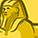Level 1
Level 1# Position2Go C CommLib Raw data in Linux terminal

Hello,

I have a query regarding the Infineon Position2Go 24GHz Radar kit. The query is as follows:

When raw data is acquired through Radar GUI and MATLAB it shows the matrix of 32*2 as a set of data. The raw data obtained are complex numbers from MATLAB. Now I want to obtain raw data using the C language in the Linux terminal. By using the C files provided in the software, only real part of raw data is acquired, even though the data obtained are 64 numbers (in listed order) just like data obtained from MATLAB. I want to know how to get the imaginary raw data in the terminal and also the sequence of data in which the data is collected from the 2 receiving antennas of IC.

Best Regards,
Walter.

1 SolutionModeratorModerator# Re: Position2Go C CommLib Raw data in Linux terminal

Hi,
The extract_raw_data.c example of the ComLib_C_Interface provided by Infineon within the P2G FW package is configured as follows
interleaved_rx = 0, data_format = RADAR_RX_DATA_COMPLEX (please refer to frame_info-interleaved_rx, and frame_info-data_format)
The radar data of multiple RX antennas (2 for P2G) is stored in consecutive data blocks, where each block holds data of one antenna.
Thus, the first 2 x 256 samples received are I1, Q1.
The index calculation of a certain data sample captured from a certain RX antenna depends on the data interleaving and on the real-complex data format.
For the extract_raw_data.c example default frame format configuration, the following code demonstrates how to access a data sample.
data_value_real = frame_start [(2 ANTENNA_NUMBER ) num_samples_per_chirp + SAMPLE_NUMBER];
data_value_imag = frame_start [(2 ANTENNA_NUMBER + 1) num_samples_per_chirp + SAMPLE_NUMBER];

ThanksModeratorModerator# Re: Position2Go C CommLib Raw data in Linux terminal

Hi,
The extract_raw_data.c example of the ComLib_C_Interface provided by Infineon within the P2G FW package is configured as follows
interleaved_rx = 0, data_format = RADAR_RX_DATA_COMPLEX (please refer to frame_info-interleaved_rx, and frame_info-data_format)
The radar data of multiple RX antennas (2 for P2G) is stored in consecutive data blocks, where each block holds data of one antenna.
Thus, the first 2 x 256 samples received are I1, Q1.
The index calculation of a certain data sample captured from a certain RX antenna depends on the data interleaving and on the real-complex data format.
For the extract_raw_data.c example default frame format configuration, the following code demonstrates how to access a data sample.
data_value_real = frame_start [(2 ANTENNA_NUMBER ) num_samples_per_chirp + SAMPLE_NUMBER];
data_value_imag = frame_start [(2 ANTENNA_NUMBER + 1) num_samples_per_chirp + SAMPLE_NUMBER];

Thanks